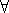## CGAL::Random_points_in_iso_box_d<Point_d>

### Definition

The class Random_points_in_iso_box_d<Point_d> is an input iterator creating points uniformly distributed in a half-open d-dimensional iso box. The default Creator is Creator_uniform_d<Kernel_traits<Point_d>::Kernel::RT, Point_d>.

### Is Model for the Concepts

InputIterator
PointGenerator

#include <CGAL/point_generators_d.h>

### Types

 typedef std::input_iterator_tag iterator_category; typedef Point_d value_type; typedef std::ptrdiff_t difference_type; typedef const Point_d* pointer; typedef Point_d reference;

### Operations

 Random_points_in_iso_box_d g ( int dim, double a, Random& rnd = default_random); g is an input iterator creating points of type Point_d uniformly distributed in the half-open d-dimensional iso box with side length 2 a, centered at the origin, i.e.p = *g: -ap.x(),p.y(),p.z() < a . d random numbers are needed from rnd for each point.